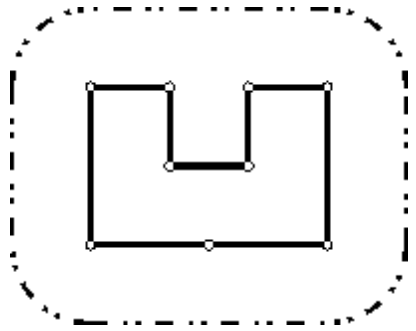# UVA1303 Wall 题解【凸包】

UVA提供各种输出格式坑

## Description

Once upon a time there was a greedy King who ordered his chief Architect to build a wall around the King’s castle. The King was so greedy, that he would not listen to his Architect’s proposals to build a beautiful brick wall with a perfect shape and nice tall towers. Instead, he ordered to build the wall around the whole castle using the least amount of stone and labor, but demanded that the wall should not come closer to the castle than a certain distance. If the King finds that the Architect has used more resources to build the wall than it was absolutely necessary to satisfy those requirements, then the Architect will loose his head. Moreover, he demanded Architect to introduce at once a plan of the wall listing the exact amount of resources that are needed
to build the wall.Your task is to help poor Architect to save his head, by writing a program that will find the minimum possible length of the wall that he could build around the castle to satisfy King’s requirements.

The task is somewhat simplified by the fact, that the King’s castle has a polygonal shape and is situated on a flat ground. The Architect has already established a Cartesian coordinate system and has precisely measured the coordinates of all castle’s vertices in feet.

## Input

The input begins with a single positive integer on a line by itself indicating the number of the cases following, each of them as described below. This line is followed by a blank line, and there is also a blank line between two consecutive inputs.
The first line of the input file contains two integer numbers $N$ and $L$ separated by a space. $N (3\le N\le 1000)$ is the number of vertices in the King’s castle, and L (1 ≤ L ≤ 1000) is the minimal number of feet that King allows for the wall to come close to the castle.

Next $N$ lines describe coordinates of castle’s vertices in a clockwise order. Each line contains two integer numbers $X_i$ and $Y_i$ separated by a space $(-10000\le X_i, Yi\le 10000)$ that represent the coordinates of i-th vertex. All vertices are different and the sides of the castle do not intersect anywhere except for vertices.

## Output

For each test case, the output must follow the description below. The outputs of two consecutive cases will be separated by a blank line.

Write to the output file the single number that represents the minimal possible length of the wall in feet that could be built around the castle to satisfy King’s requirements. You must present the integer number of feet to the King, because the floating numbers are not invented yet. However, you must round the result in such a way, that it is accurate to 8 inches (1 foot is equal to 12 inches), since the King will not tolerate larger error in the estimates.

## Sample Input

1
9 100
200 400
300 400
300 300
400 300
400 400
500 400
500 200
350 200
200 200

## Sample Output

1628

## 题意：

给定一些点，求出包围这些点且与这些点的距离不小于给定的$L$的最短连续轮廓长度。

## 题解：

一开始还根据两条边之间的夹角来判断弧长，后来发现做麻烦了。

直接求出凸包再加上$2\pi L$就可以了。

我为什么挂在了2.0*pi和输出格式上

#include<cstdio>
#include<cstring>
#include<cmath>
#include<algorithm>
#define db double
#define eps 1e-8
using std::sort;
const db pi=acos(-1);
struct vct
{
db x,y;
vct(db x,db y)
{
this->x=x;
this->y=y;
}
vct(){}
friend vct operator +(vct a,vct b)
{return vct(a.x+b.x,a.y+b.y);}
friend vct operator -(vct a,vct b)
{return vct(a.x-b.x,a.y-b.y);}
friend db operator &(vct a,vct b)//点积
{return a.x*b.x+a.y*b.y;}
friend db operator *(vct a,vct b)
{return a.x*b.y-a.y*b.x;}
friend bool operator <(vct a,vct b)
{
if(fabs(a.x-b.x)>eps)
return b.x-a.x>-eps;
return b.y-a.y>-eps;
}
}p,stk;
db dis(vct a)
{
return sqrt(a.x*a.x+a.y*a.y);
}
int tp=0;
int main()
{
int T;
scanf("%d",&T);
while(T--)
{
int n;
db r;
scanf("%d%lf",&n,&r);
for(int i=1;i<=n;++i)
scanf("%lf%lf",&p[i].x,&p[i].y);
sort(p+1,p+1+n);
stk[++tp]=p;
for(int i=2;i<=n;++i)
{
while(tp>=2&&(stk[tp]-stk[tp-1])*(p[i]-stk[tp])<-eps)
--tp;
stk[++tp]=p[i];
}
stk[++tp]=p[n-1];
for(int i=n-2;i>=1;--i)
{
while(tp>=2&&(stk[tp]-stk[tp-1])*(p[i]-stk[tp])<-eps)
--tp;
stk[++tp]=p[i];
}
db sum=0.0;
for(int i=2;i<=tp;++i)
sum+=dis(stk[i]-stk[i-1]);
printf("%.0lf\n",sum+2.0*pi*r);
if(T)
puts("");
tp=0;
}
return 0;
}

### 3 说点什么0 Followers

Most reacted comment
0 Comment authors
Recent comment authors
Subscribe[…] Information on that Topic: wjyyy.top/2845.html […]wpDiscuz